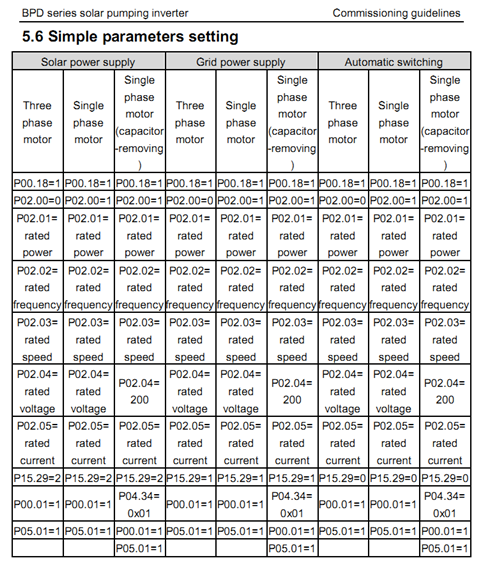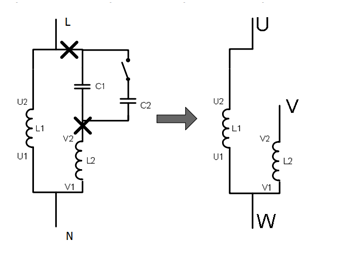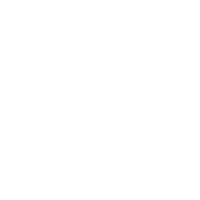# NEWS

### FAQ about INVT BPD Series Solar Pump Inverter

Release time：2020-04-10　Click amount： 2726
Share

Firstly, read the operation manual, and then set the parameters as the last page in the manual, select the suitable column refer to the real situation.Q1: Keypad display AL-S, and system stop running?

A: AL-S is weak light alarm, means the current sunshine is not enough to drive the pump, result in the running frequency Hz is less than lower limit: (lower limit: P15.05*P00.03)

Conditions of AL-S:

a. Running frequency is less than P15.05*P00.03, and keep more than P15.20 time.

b. Current PV output voltage (P18.01) is less than under voltage point.

(1HP: 60V, 2HP/3HP: 80V)

If current condition meets a or b, BPD will display AL-S alarm.

If P15.29=0, the system will switch to AC power input channel.

Q2：Single phase pump cannot start?

A. If removed the capacitors? Please remove the capacitors as the following photo, and make sure the resistor value U&V is the biggest OHM, U&W is the smallest value.

After the capacitors are removed, need to set P04.34=01. ( when set this value, Press SHIFT key can change the setting bit.)Q3: The keypad display OL2 or OC1 code when start？

A: 1. Please change the ACC time P00.11=5.0 seconds, test it again.

2. If the pump is single phase, remove the capacitors as the Q2.

3. If the pump running direction is reverse, switch U and W if the pump is 3phase, or set P00.13=1;

If the pump is single phase and capacitors are removed, set P04.34=11.

4. SetP02.01~P02.05 as the pump nameplate.

5. The BPD power rating is lower, suggest to replace a bigger power rating one.

Q4: BPD display At-F or At-L, and system stop running？

A: 1. At-F or At-L means full water level alarm, or empty water level alarm. Check the sensor.

2. Normally the full water level sensor in the tank connect S2 and COM, Empty water level sensor in the well connect S3 and COM. S2 signal result in At-F, S3 signal result in At-L.

3. Check the sensor contact, if the NO or NC can work well.

4. Commissioning the sensor as the right document.

Q5: BPD cannot start automatically in the morning?

A: Check P15.29=0 or not. If equals 0, means it can switch between AC&PV.

B：Check the LOCAL/REMOT LED, if the LED is off, need to set: P00.01=1, P05.01=1.

Q6: Cannot switch between AC&PV?

A: 1. Check the parameters, P15.29 must equals0.

2. AC/PV switch logic is:

PVAC:

a. Refer to Q1 AL-S condition;

b. PV output voltage (P18.01) is less than P15.30,

The relationship between a and b is OR

ACPV: PV output voltage is bigger than P15.31, after P15.21 time, switch to PV.

Q7: The system switch between PV & AC frequently?

A: 1. Check the value of P15.30&P15.31, if the value is suitable, generally P15.31 is bigger 60V than P15.30, suggest to increase P15.31.

2. Check if the lower limit percentage P15.05 is too high.

Q8: How to set running frequency lower limit?

A: 1. Lower limit frequency is calculate as this: P15.05*P00.03. Default value is: P00.03*P15.05=50Hz*20%=10Hz. If the running frequency is less than 10Hz, and keep 15.20 seconds, will display AL-S, or switch to AC.

Q9: Pump is running, but there is no water in the pipe?

A: 1. Check the pump running direction, P00.13=1 can change the direction.

2. Pump head and the frequency is proportional to the square, according to the current operating frequency, please calculate the current head is satisfied or not.

Q10: How to check the PV output voltage?

A: 1. P18.01 is current PV output voltage.

Q11：How could I know the current working status is AC or PV?

A: 1. P18.10=0: PV channel working

P18.10=1: AC channel working.

Q12: How to check the current PV input power?

A: P18.07 is current input power, unit is KW.

Q13： I check P18.07 reach the rated pump power, but the running frequency is less than 50Hz?

A: The reason is the pump efficiency, generally the pump efficiency is as the followings: Suggest the solar power is ≥ ( pump power / efficiency)

 Pump η ≤0.75kW 0.65 ≤2.2kW 0.7 ≤5.5kW 0.75 ≤18.5kW 0.8 ≤55kW 0.85

Q14: How to configure the solar panel?

A: 1. All the panels in series Vmp > 100V.

2. All the panels in series Voc < 450V.

3. Solar panel power > ( pump power / efficiency)

Q15: What need to pay attention to if I drive single phase pump?

A: BPD output rated current A >  1.4 times of pump current AMP.

After remove the capacitor, P04.35=1.0~1.4, P04.34=01.

Q16: Why the output water flow is lower, after I remove the capacitor?

A: As the single-phase motor contains the starting capacitor, the start-up capacitor is designed to generate a 90-degree phase difference between the primary and secondary windings of the single-phase motor, resulting in a rotating magnetic field to rotate the motor. The size of the starting capacitor is generally designed according to the frequency 50Hz, but when the single-phase motor frequency control, the frequency will not always work at 50Hz, it is difficult to produce rotating magnetic field, the common method is to remove the single-phase motor start and run Capacitor, the use of two-phase control method.

The use of the method of removing the capacitor, the software algorithm improvements, it is necessary to ensure that limit the starting current, but also to ensure that the starting torque, so the final output voltage than the direct start voltage amplitude is low, resulting in load capacity decreased.

Related recommendations